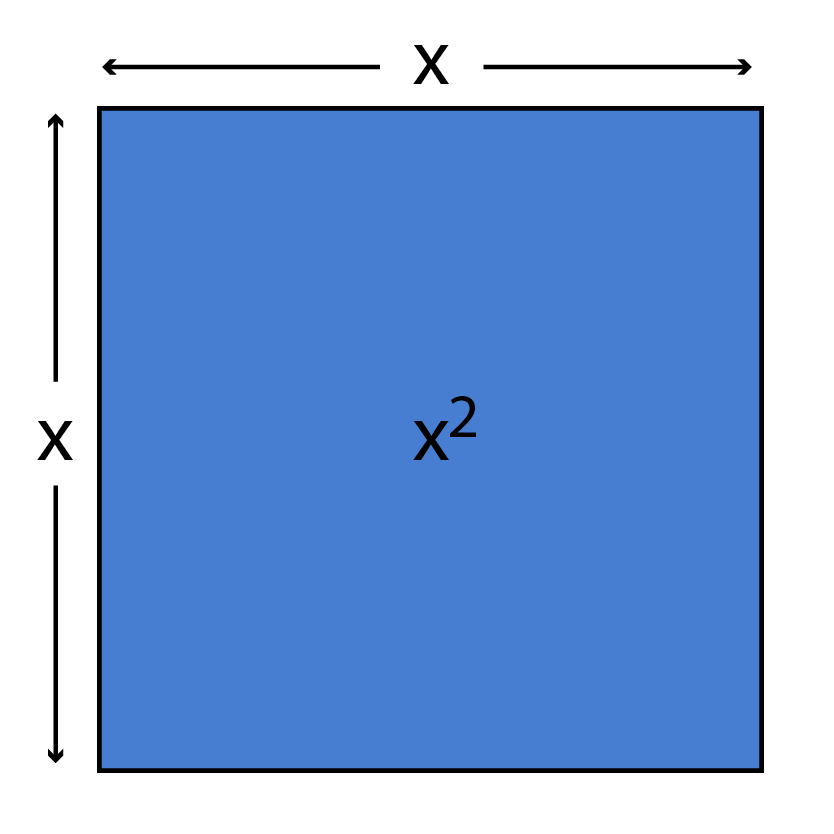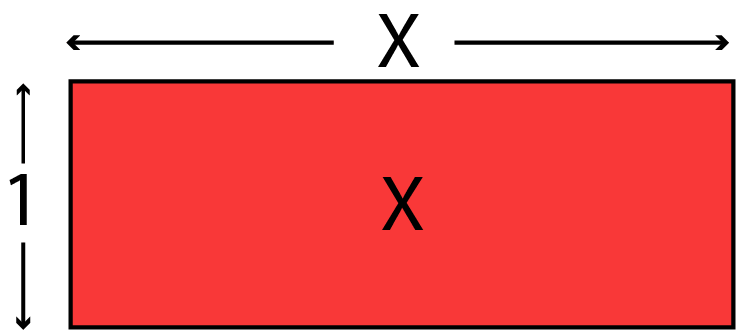Algebraic Identity (a²-b²)

## Objective

To verify the algebraic identity (a² - b²)= (a+b)(a-b)

## Algebraic Identity

• An Algebraic identity is an equality, which is true for all values of the variables in the equality.
• The algebraic equations which are valid for all values of variables in them are called algebraic identities.
• In this way, algebraic identities are used in the computation of algebraic expressions and solving different polynomials.
• Algebraic identities are also used for the factorization of polynomials.

## Square

###A square is a regular quadrilateral, which means that it has four equal sides and four equal angles of 90 degrees each.

## Area of a Square

The product of the length of each side itself.

Formula : Area = side²=x²

## Rectangle

###Rectangle is a quadrilateral with four right angles and opposite sides are equal.

## Area of a Rectangle

The product of its length and breadth

Formula : Area = length * breadth = x * 1